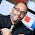## Tuesday, March 22, 2011

### How to calculate R-squared for a decision tree model

A client recently wrote to us saying that she liked decision tree models, but for a model to be used at her bank, the risk compliance group required an R-squared value for the model and her decision tree software doesn't supply one. How should she fill in the blank? There is more than one possible answer.

Start with the definition of R-squared for regular (ordinary least squares) regression. There are three common ways of describing it. For OLS they all describe the same calculation, but they suggest different ways of extending the definition to other models. The calculation is 1 minus the ratio of the sum of the squared residuals to the sum of the squared differences of the actual values from their average value.

The denominator of this ratio is the variance and the numerator is the variance of the residuals. So one way of describing R-squared is as the proportion of variance explained by the model.

A second way of describing the same ratio is that it shows how much better the model is than the null model which consists of not using any information from the explanatory variables and just predicting the average. (If you are always going to guess the same value, the average is the value that minimizes the squared error.)

Yet a third way of thinking about R-squared is that it is the square of the correlation r between the predicted and actual values. (That, of course, is why it is called R-squared.)

Back to the question about decision trees: When the target variable is continuous (a regression tree), there is no need to change the definition of R-squared. The predicted values are discrete, but everything still works.

When the target is a binary outcome, you have a choice. You can stick with the original formula. In that case, the predicted values are discrete with values between 0 and 1 (as many distinct estimates as the tree has leaves) and the actuals are either 0 or 1. The average of the actuals is the proportion of ones (i.e. the overall probability of being in class 1).  This method is called Efron's pseudo R-squared.

Alternatively, you can say that the job of the model is to classify things.  The null model would be to always predict the most common class. A good pseudo R-squared is how much better does your model do? In other words, the ratio of the proportion correctly classified by your model to the proportion of the most common class.

There are many other pseudo R-squares described on a page put up by the statistical consulting services group at UCLA.

1.I should perhaps add that just because it is possible to think of ways to calculate an R-squared statistic for a decision tree doesn't mean that there is much point in doing so. Direct measures of how well the model is doing what it is supposed to do are generally more helpful: lift, classification error, root mean squared error, and the like.

2.It seems that the original challenge stems from a lack of public education in current machine learning techniques. R measure is mainly used in statistics and not really applicable to a decision tree. In a world where data mining is more prevalent we need to educate and inform more about how it works and how to apply it in a business setting. If the people needing this information are more educated on data mining and machine learning practices and metrics then they might be more apt to accept the model without trying to apply a qualitative metric that while valid, does not fit the model.

3.It seems that the original challenge stems from a lack of public education in current machine learning techniques. R measure is mainly used in statistics and not really applicable to a decision tree. In a world where data mining is more prevalent we need to educate and inform more about how it works and how to apply it in a business setting. If the people needing this information are more educated on data mining and machine learning practices and metrics then they might be more apt to accept the model without trying to apply a qualitative metric that while valid, does not fit the model.

Your comment will appear when it has been reviewed by the moderators.